# Samacheer Kalvi Books: Tamilnadu State Board Text Books Solutions

## Samacheer Kalvi 7th Maths Percentage and Simple Interest Notes PDF Download: Tamil Nadu STD 7th Maths Percentage and Simple Interest NotesSamacheer Kalvi 7th Maths Percentage and Simple Interest Notes PDF Download: Tamil Nadu STD 7th Maths Percentage and Simple Interest Notes

## Samacheer Kalvi 7th Maths Percentage and Simple Interest Notes PDF Download

We bring to you specially curated Samacheer Kalvi 7th Maths Percentage and Simple Interest Notes PDF which have been prepared by our subject experts after carefully following the trend of the exam in the last few years. The notes will not only serve for revision purposes, but also will have several cuts and easy methods to go about a difficult problem.

 Board Tamilnadu Board Study Material Notes Class Samacheer Kalvi 7th Maths Subject 7th Maths Chapter Percentage and Simple Interest Format PDF Provider Samacheer Kalvi Books

## How to Download Samacheer Kalvi 7th Maths Percentage and Simple Interest Notes PDFs?

2. Click on the Samacheer Kalvi 7th Maths Notes PDF.
3. Look for your preferred subject.
4. Now download the Samacheer Kalvi 7th Maths Percentage and Simple Interest notes PDF.

## Tamilnadu Samacheer Kalvi 7th Maths Solutions Term 3 Chapter 2 Percentage and Simple Interest Intext Questions

Exercise 2.1
Try These (Text book Page No. 28)

Question 1.
Find the percentage of children whose scores fall in different categories given in table below.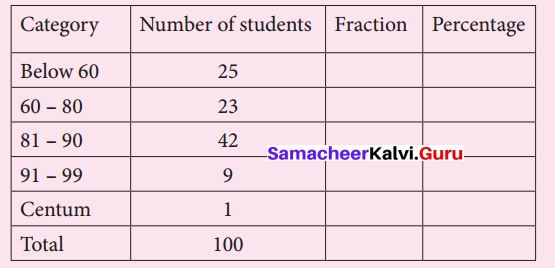Solution: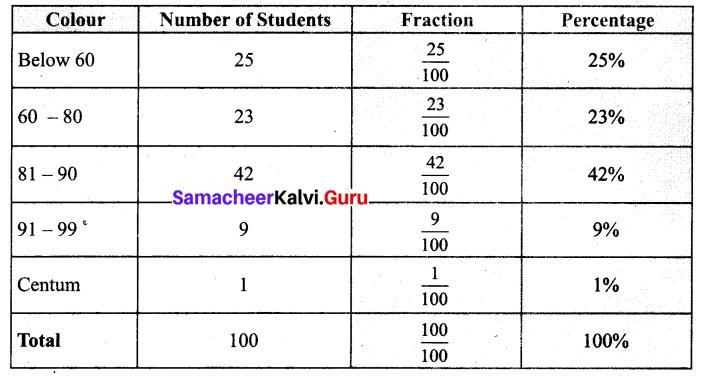Try These (Text book Page No. 29)

Question 1.
There are 50 students in class VII of a school. The number of students involved in these activities are :
Scout: 7
Red Ribbon Club : 6
Junior Red Cross : 9
Green Force : 3
Sports : 14
Cultural activity : 11
Find the percentage of students who involved in various activities.
Solution: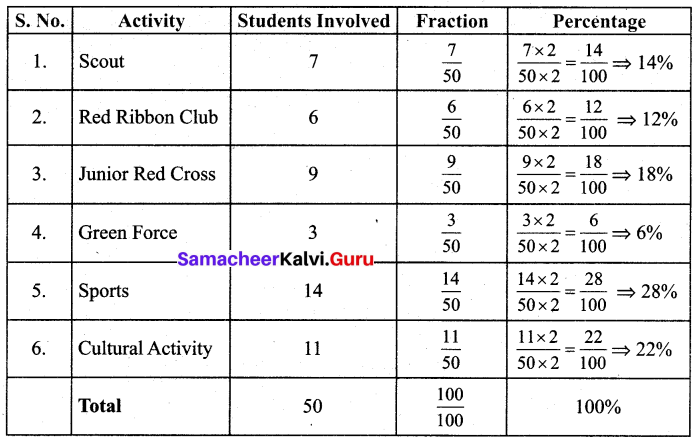Try These (Text book Page No. 30)

Question 1.
Convert the fractions as percentage.
(i) 120
(ii) 1325
(iii) (i) 4550
(iv) 185
(v) 2710
(vi) 7290
Solution:
(i) 120
= 120 × 100100
= 120 × 100 %
= 5 %

(ii) 1325
= 1325 × 100100
= 1325 × 100 %
= 52 %

(iii) 4550
= 4550 × 100100
= 4550 × 100 %
= 90 %

(iv) 185
= 185 × 100100
= 1850 × 100 %
= 360 %

(iv) 2710
= 2710 × 100100
= 2710 × 100 %
= 270 %

(iv) 2710
= 2710 × 100100
= 2710 × 100 %
= 270 %

(vi) 7290
= 7290 × 100100
= 7290 × 100 %
= 80 %

Question 2.
Convert the following percentage as fractions.
(i) 50%
(ii) 75%
(iii) 250%
(iv) 30 15 %
(v) 720 %
(vi) 90 %
Solution:
(i) 50 %
= 50100
= 510
= 12

(ii) 75 %
= 75100
= 34

(iii) 250 %
= 250100
= 2510
= 52

(iv) 30 15 %
= 3015100=(1515)100
= 151500

(v) 720 %
= 720100=720×100
= 72000

(vi) 90 % = 90100 = 910

Think (Text book Page No. 32)

Question 1.
What is the difference between 0.01 and 1%.
Solution:
0.01 = 1100 = 1%
0.01 and 1% are the same.

Question 2.
In a readymade shop there will be a board showing upto 50% off. Most of the people will realize that everything is half of its original price, Is that true?
Solution:
No. Only some of them are half of its original price.

Exercise 2.2
Try These (Text book Page No. 33)

Question 1.
Convert these decimals to percentage.
(i) 0.25
(ii) 0.07
(iii) 0.8
(iv) 0.375
(v) 3.75
Solution:
(i) 0.25
= 25100 = 25 %

(ii) 0.07
= 7100 = 7 %

(iii) 0.8
= 80100 = 80 %

(iv) 0.375
= 3751000
= 37510 × 1100
= 37.5 %

(v) 3.75
= 375100 = 375 %

Try These (Text book Page No. 34)

Question 1.
Write these percentage as decimals
(i) 3 %
(ii) 25 %
(iii) 80 %
(iv) 67 %
(v) 17.5 %
(vi) 135 %
(vii) 0.5 %
Solution:
(i) 3 %
= 3100 = 0.03

(ii) 25 %
= 25100 = 0.25

(iii) 80 %
= 80100 = 0.8

(iv) 67 %
= 67100 = 0.67

(v) 17.5 %
= 17.5100 = 0.175

(vi) 135 %
= 135100 = 1.35

(vii) 0.5 %
= 0.5100 = 0.005

Exercise 2.3
Try These (Text book Page No. 38)

Question 1.
Level of water in a tank is increased from 35 litres to 50 litres in 2 minutes, what is the Percentage of increase?
Solution:
Level of water in the tank originally = 35 litres.
Increase in the water level = amount of change = 50 – 35 = 15 litres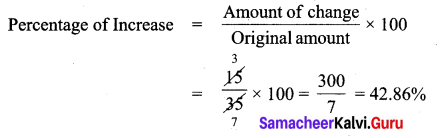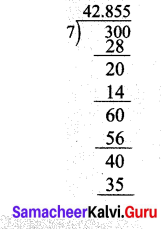Exercise 2.4
Try These (Text book Page No. 41)

Question 1.
Arjun borrowed a sum of ₹ 5,000 from a bank at 5% per annum. Find the interest and amount to be paid at the end of three year.
Solution:
Here Principal (P) = ₹ 5,000
Rate of interest (r) = 5 % Per annum
Time (n) = 3 years
Simple Interest I = pnr100
= 5000×3×5100
= ₹ 750
Amount to be paid A = P + I = ₹ 5,000 + ₹ 750 = ₹ 5,750
I = ₹ 750 ; A = ₹ 5,750

Question 2.
Shanti borrowed ₹ 6,000/- from a Bank for 7 years at 12 % per annum. What amount will clear off her debt?
Solution:
Here principal (P) = ₹ 6,000
Rate of Interest (r) = 12 % Per annum
Time (n) = 4 Years
Simple Interest (I) = pnr100 =
= 6000×7×12100
I = ₹ 5,040
Amount to be paid A = P + I = 6,000 + 5,040 = ₹ 11,040

Think (Text book Page No. 43)

Question 1.
In simple interest, a sum of money doubles itself in 10 years. In how many years it will get triple itself.
Solution:
Let the Principal be P and Rate of interest be r % per annum.
Here the number of years n = 10 years
Given in 10 years P becomes 2 P.
A = P + I
After 2 years A = 2P
i.e. 2P = P + I
2P – P = I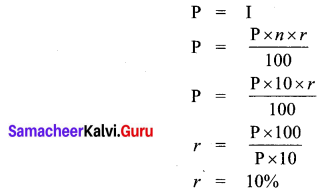Now if the amount becomes triple then A = P + I = 3P
3P = P + I
3P – P = I
2P = I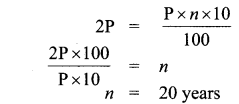∴ After 20 years the amount get tripled.

## How to Prepare using Samacheer Kalvi 7th Maths Percentage and Simple Interest Notes PDF?

Students must prepare for the upcoming exams from Samacheer Kalvi 7th Maths Percentage and Simple Interest Notes PDF by following certain essential steps which are provided below.

• Use Samacheer Kalvi 7th Maths Percentage and Simple Interest notes by paying attention to facts and ideas.
• Pay attention to the important topics
• Refer TN Board books as well as the books recommended.
• Correctly follow the notes to reduce the number of questions being answered in the exam incorrectly
• Highlight and explain the concepts in details.

## Frequently Asked Questions on Samacheer Kalvi 7th Maths Percentage and Simple Interest Notes

#### How to use Samacheer Kalvi 7th Maths Percentage and Simple Interest Notes for preparation??

Read TN Board thoroughly, make separate notes for points you forget, formulae, reactions, diagrams. Highlight important points in the book itself and make use of the space provided in the margin to jot down other important points on the same topic from different sources.

#### How to make notes for Samacheer Kalvi 7th Maths Percentage and Simple Interest exam?

Read from hand-made notes prepared after understanding concepts, refrain from replicating from the textbook. Use highlighters for important points. Revise from these notes regularly and formulate your own tricks, shortcuts and mnemonics, mappings etc.
Share: